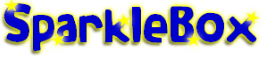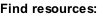﻿ Primary School Subtraction Activities and Games resources - SparkleBox

HOME > Maths > Calculations > Subtraction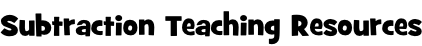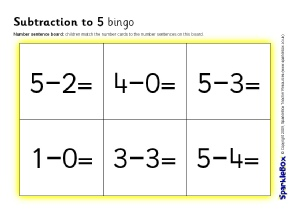Subtraction to 5 Bingo (SB2876)

A set of printable bingo boards and cards with simple subtraction number sentences with answers to 5.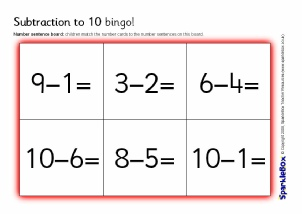Subtraction to 10 Bingo (SB1809)

A set of printable bingo boards and cards with simple subtraction number sentences with answers to 10.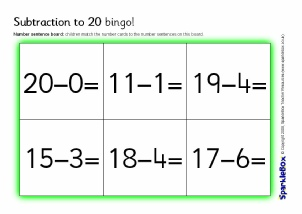Subtraction to 20 Bingo (SB1822)

A set of printable bingo boards and cards with simple subtraction number sentences with answers from 10-20.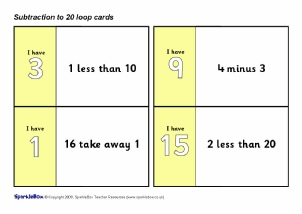Subtraction to 20 Loop Cards (SB2170)

A set of printable loop cards featuring various subtraction problems with numbers to 20.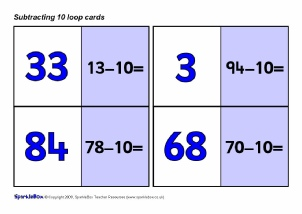Subtract 10 Loop Cards (SB2316)

A set of printable loop cards with subtract 10 number sentences.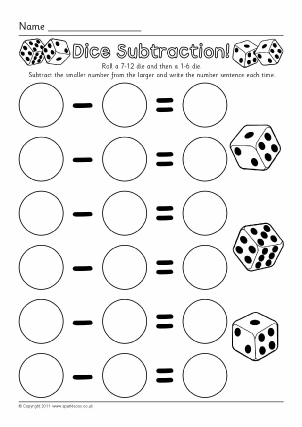Dice Subtraction Worksheets (SB7331)

Simple worksheets where pupils roll a 7-11 die and a 1-6 die, subtract the smaller number from the larger and write the number sentence.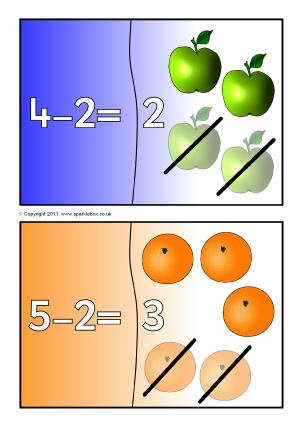Subtraction Mini Jigsaws (SB4864)

A set of printable 2-piece jigsaws where children match together the number sentence with the corresponding total.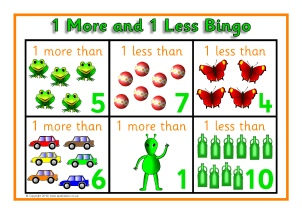1 more and 1 less Bingo to 10 (SB3134)

A set of printable bingo boards and cards where children match number cards that are 1 more or 1 less.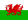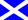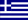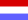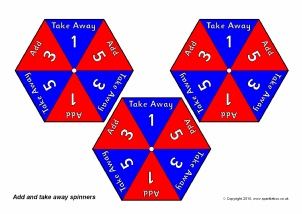Add and Take Away Spinner Templates (SB3221)

Printable pencil spinners for use in tabletop games in colour and black and white.  Ideal for use with the Phase 6 ‘Spinners’ activity outlined in the DCSF ‘Numbers and Patterns’ publication (PDF page 159).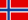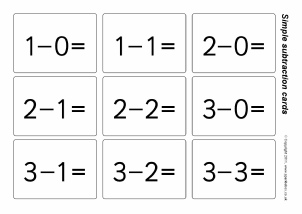Simple Subtraction Flash Cards - Numbers to 20 (SB5663)

Simple flash cards featuring subtraction number sentences (without answers) that use numbers to 20.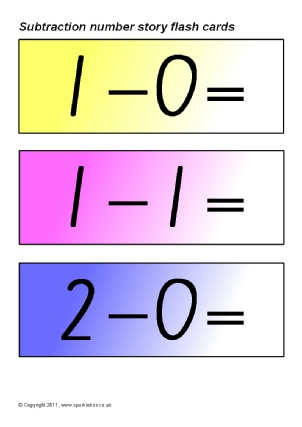Subtraction Number Story Flash Cards (SB4421)

Simple flash cards showing subtraction number sentences for numbers up to 10.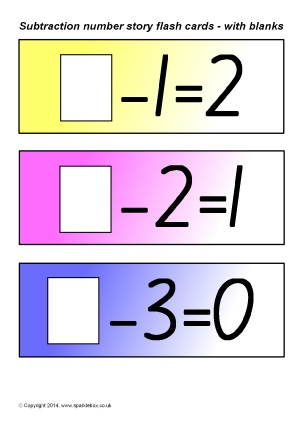Subtraction Missing Number Story Flash Cards (1 to 10) (SB10344)

Simple flash cards showing number sentences with blanks for making numbers 1 to 10.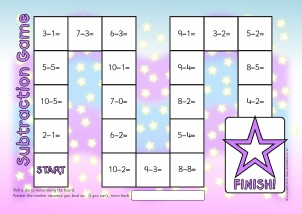Subtraction Board Game (Numbers to 10) (SB7265)

A basic board game where children move around the board and answer the subtraction number sentences.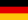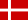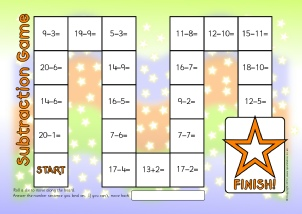Subtraction Board Game (Numbers to 20) (SB7266)

A basic board game where children move around the board and answer the subtraction number sentences.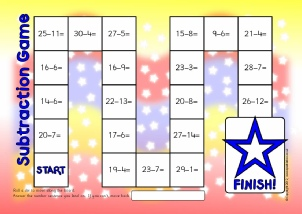Subtraction Board Game (Numbers to 30) (SB7268)

A basic board game where children move around the board and answer the subtraction number sentences.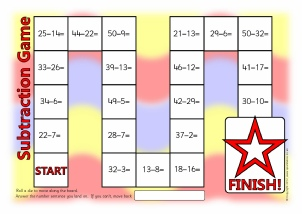Subtraction Board Games (Numbers to 50) (SB7269)

Simple board games where children move around the board and answer the subtraction number sentences.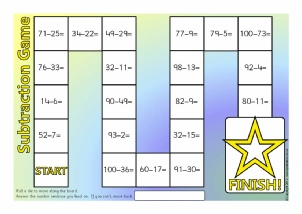Subtraction Board Games (Numbers to 100) (SB7271)

Simple board games where children move around the board and answer the subtraction number sentences.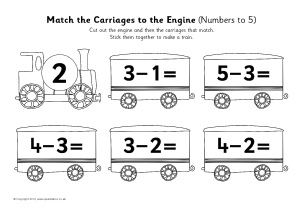Train Subtraction Cut and Stick Activity (numbers to 5) (SB7053)

A simple cut and stick activity where children cut out the engines and carriages that match and stick them together to make a train.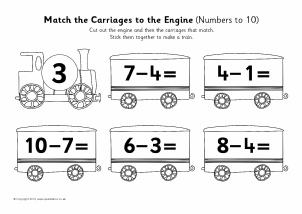Train Subtraction Cut and Stick Activity (Numbers to 10) (SB7054)

A simple cut and stick activity where children cut out the engines and carriages that match and stick them together to make a train.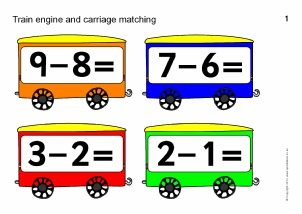Train Subtraction Matching Activity (Numbers to 10) (SB7055)

Printable train engines with numbers 0 to 10 and carriages with number sentences.  Create a matching activity where children match the carriages to the corresponding engines.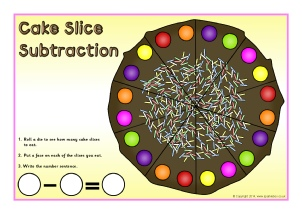Cake Slice Subtraction Mats (SB10515)

Printable desktop activity mats where children roll a dice and ‘eat’ the cake slices and write the subtraction number sentence.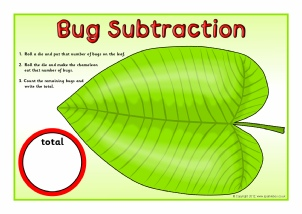Bug Subtraction Mats (SB7047)

Printable desktop activity mats where children roll a die and put bugs on a leaf and then roll again for the chameleon to eat some.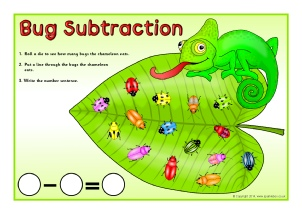Bug Subtraction Mats 2 (SB10218)

Printable desktop activity mats where children roll a die, cross out the bugs that the chameleon eats and then write the number sentence.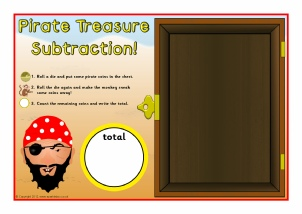Pirate Treasure Subtraction Mats (SB8246)

Printable desktop activity mats where children roll a dice and place coins in the pirate chest.  They roll again for the monkey to sneak some away, then write the total or number sentence.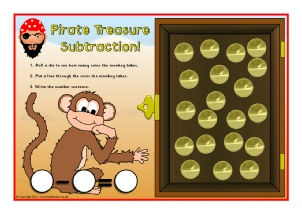Pirate Treasure Subtraction Mats 2 (SB10221)

Printable desktop activity mats where children roll a die, cross out the coins that the monkey takes and then write the number sentence.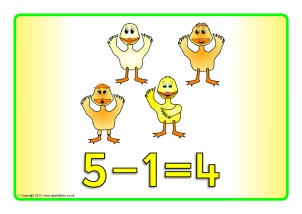Five Little Ducks Number Sentence Posters (SB4818)

A set of printable posters with subtraction number sentences to accompany the well-known counting rhyme.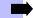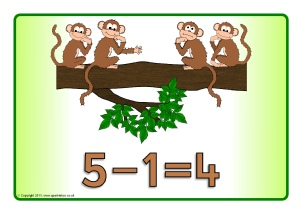Five Little Monkeys Number Sentence Posters (SB4817)

A set of printable posters with subtraction number sentences to accompany the well-known counting rhyme.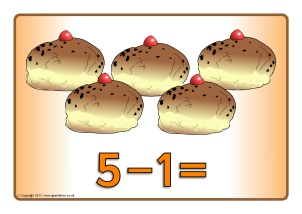Five Currant Buns Number Sentence Posters (SB4830)

A set of printable posters with subtraction number sentences to accompany the well-known counting rhyme.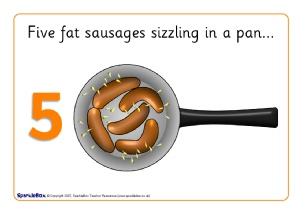Five/Ten Fat Sausages Number Sentence Posters (SB4858)

A set of printable posters with subtraction number sentences to accompany the well-known counting rhyme.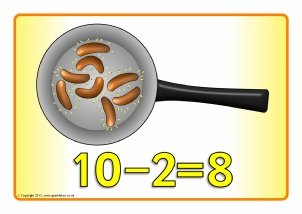Ten Fat Sausages (Taking 2) Number Sentence Posters (SB4859)

A set of printable posters with subtraction number sentences to accompany the well-known counting rhyme.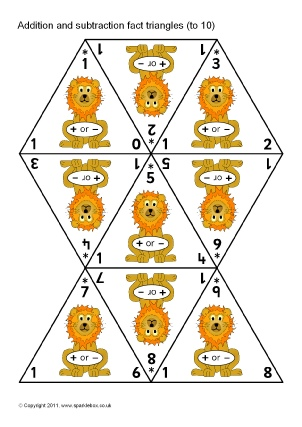Addition and Subtraction Fact Triangles (to 10) (SB6107)

Printable addition/subtraction fact triangles with numbers up to 10.  Featuring a selection of cartoon animal characters.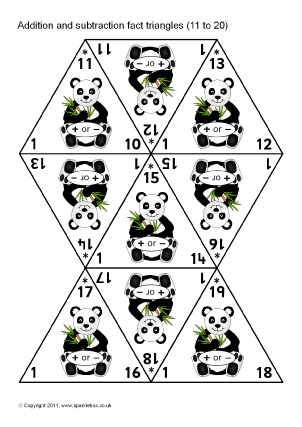Addition and Subtraction Fact Triangles (10-11) (SB6140)

Printable addition/subtraction fact triangles with numbers 11 to 20.  Featuring a selection of cartoon animal characters.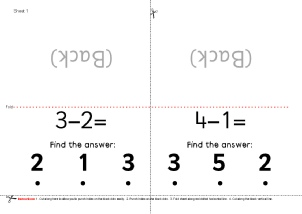Subtraction Hole-Punch Cards Activity Numbers to 10) (SB7381)

Create an activity where children read and answer the number sentence on a fold-over card, mark a dot through the corresponding hole and check using the pictures beneath.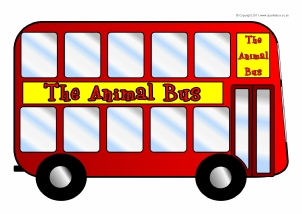A great activity to reinforce addition or subtraction.  There are ten windows on the bus.  Children can place different animals ‘window’ cards onto and off the bus.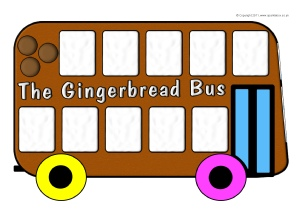A great activity to reinforce addition or subtraction.  There are ten windows on the bus.  Children can place gingerbread man/woman ‘window’ cards onto and off the bus.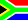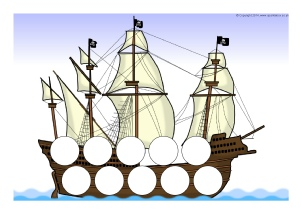A great activity to reinforce addition or subtraction.  There are ten spaces on the ship.  Children can place pirates onto and off the ship for simple addition and subtraction.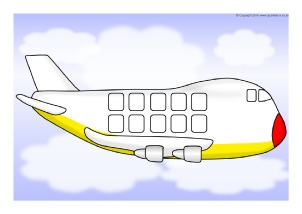A great activity to reinforce addition or subtraction.  Aeroplanes with five/ten spaces on them.  Children can place passengers onto and off the plane for simple addition and subtraction.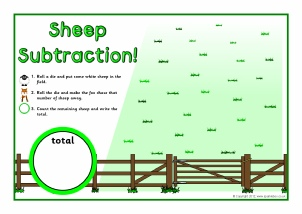Sheep Subtraction Mats (SB7384)

Printable desktop activity mats where children roll a dice and place sheep in the field.  They roll again for the fox to chase some away, then write the total or number sentence.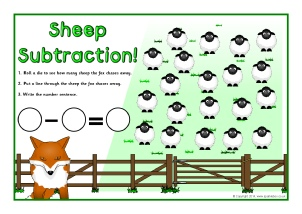Sheep Subtraction Mats 2 (SB10222)

Printable desktop activity mats where children roll a die, cross out the sheep the fox chases away and write the number sentence.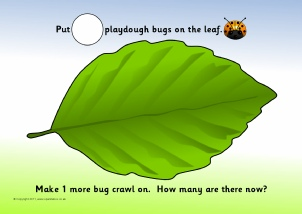1 more and 1 less Playdough Mats (SB5223)

A set of A4-size playdough mats where children make 1 more or take 1 away.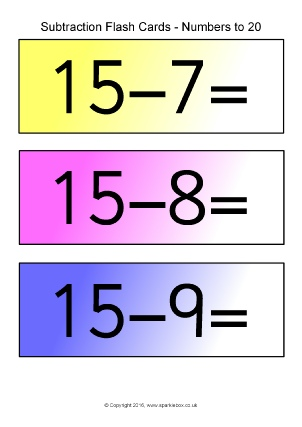Subtraction Number Sentence Flash Cards (SB11564)

Simple flash cards showing subtraction number sentences that use numbers 20 (without solutions).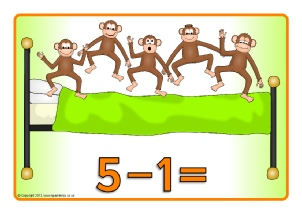Five Little Monkeys Jumping on the Bed Number Sentence Posters (SB9277)

A set of printable posters with subtraction number sentences to accompany the well-known counting rhyme.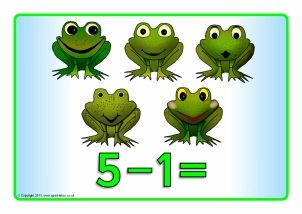Five Little Speckled Frogs Number Sentence Posters (SB4846)

A set of printable posters with subtraction number sentences to accompany the well-known counting rhyme.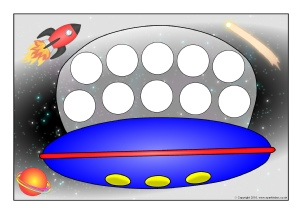A great activity to reinforce addition or subtraction.  There are ten spaces on the flying saucer.  Children can place aliens onto and off the flying saucer for simple addition and subtraction.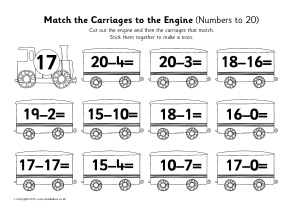Train Subtraction Cut and Stick Activity (Numbers to 20) (SB11709)

A simple cut and stick activity where children cut out the engines and carriages that match and stick them together to make a train.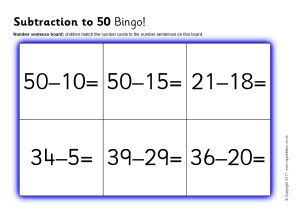Subtraction to 50 Bingo (SB11801)

A set of printable bingo boards and cards with simple subtraction number sentences with numbers and solutions to 50.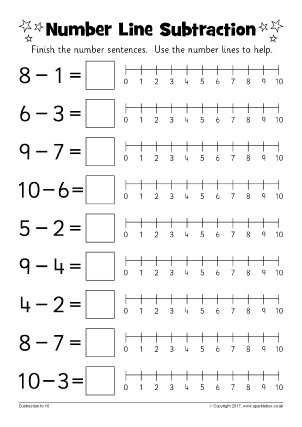Number Line Subtraction Worksheets (SB12219)

Simple worksheets with subtraction number sentences to 10 and 20. The sheets include number lines for children to use to help with the subtraction.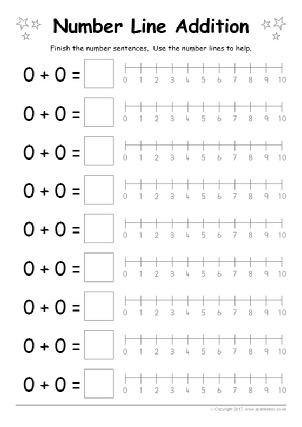Editable Number Line Addition/Subtraction Worksheets (SB12218)

Editable worksheet templates for Microsoft Word featuring number lines for children to use to help them complete the number sentences. Customise the text yourself and add your own addition or subtraction sums to suit your pupils' needs.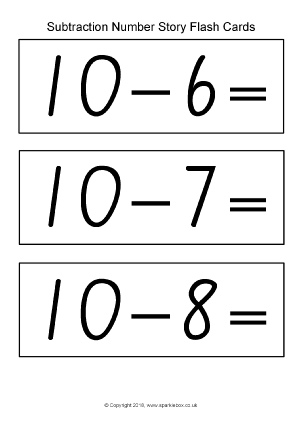Subtraction Number Story Flash Cards – Black and White (SB12442)

Simple flash cards showing subtraction number sentences for numbers up to 10.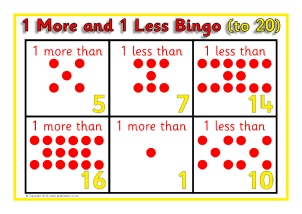1 more and 1 less Bingo to 20 (SB3146)

A set of printable bingo boards and cards where children match number cards that are 1 more or 1 less.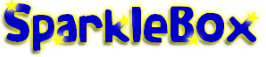New Literacy Maths Signs and Labels Class Management Special Needs Other Topics